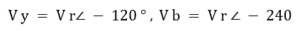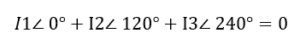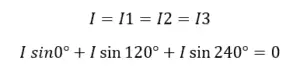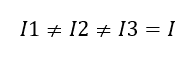The load in a poly phase circuit can be classified into two categories are

### Balance circuit:

The circuit said to be balance circuit then, the voltage of each phase should be in same magnitude and the equal phase difference.

Example:

Take three phase balance circuit… the i.e., the magnitude of the voltage is equal and phase difference is 120 deg each other. here B phase lagging Y phase by 120° and Y phase lagging R by 120°.The three current flow through load circuit are I1 , I2 and I3.

Here..All are equal in magnitude and having equal phase difference. hence the equal magnitude of the current flow through the circuit can be written asLet’s prove it…take LHS

That’s why we can say it as balance circuit.

You can take six phase circuit also…the same way you can prove as balance circuit.

Factor affecting balance circuit:

• Load condition.i.e inductive load winding inductance varies according to the coil
• Magnitude of the voltage… if the voltage magnitude varies then the current flow also varies
• Single phase load should not be given.
• The phase difference should be equal.
• The system should not have any fault such as symmetrical fault and unsymmetrical fault.